Study Guide

# Congruent and Similar Solids

## Congruent and Similar Solids

By now, we've earned quite a bit of street cred working with surface area and volumes. You could throw us any shape and we'd give you its surface area, volume, and even its pants size.

What we need now is a way to relate everything together. We can compare and contrast volumes and surface areas all the livelong day, but we'll only get caught in a web of formulas and confusion.

Instead, we'll take a look at how shapes are similar, congruent, or neither.

Similar solids have the same shape but not the same size. If we put their Facebook profile pictures side by side they wouldn't look similar, but all it takes is a comparison of their edges.

If the ratio of measures of the pyramids is the same for all the different measures in both solids, the two are similar.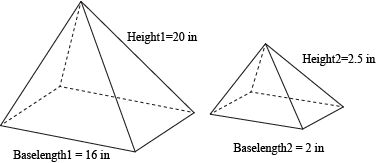The ratio of the heights should equal the ratio of the base lengths. Basically, every measurement should have the same ratio, called the scale factor.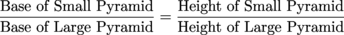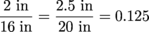In this case, the scale factor is 0.125. Let's give it a go.

### Sample Problem

Are the two basketballs below similar or not? If they are, what is their scale factor?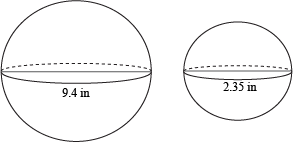Comparing their diameters, we get: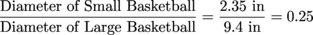Yes, the two are similar with a scale factor of 0.25. Actually since a sphere's only important measurement is its radius (since diameter, circumference, and pretty much everything else depends on the radius anyway), all spheres are similar to each other. Like circles, remember?

Two solids are congruent only if they're clones of each other. That means their scale factor has to be exactly 1. In other words, all their angles, edges, and faces are congruent. Even their volumes have to be equal. Harsh rules, man.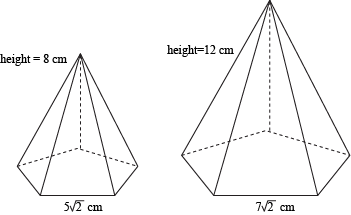So is this pair of pyramids congruent, similar, or neither? Please contain your enthusiasm.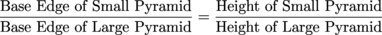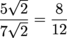0.714 ≠ 0.667

Since the proportions don't match, the solids are not similar and there's no scale factor. That means we don't have to worry about slant height. If the base edges and heights had the same ratio, we'd have to check the slant height, too. It's all or nothin'.

### Sample Problem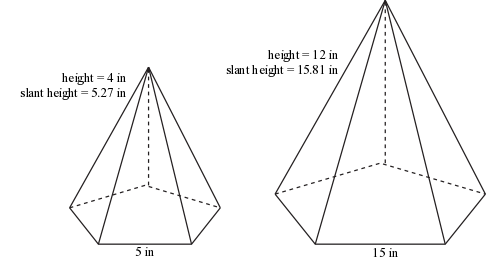What about these guys? Are they similar or not?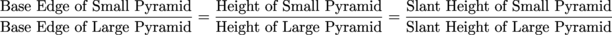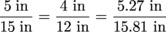0.333 = 0.333 = 0.333

Yep. The pyramids have a scale ratio of 1:3, or one third. The measurements of the smaller pyramid are one-third the size of the larger one, but what about the surface areas and volumes?

We know how to calculate surface area already (we spent three chapters on it—we're beat!), so we'll speed past that part. The surface areas of the pyramids are about 109 in2 for the smaller one and 980.7 in2 for the larger one. The ratio of the surface areas isn't 1:3. It's 1:9. How peculiar.

If we calculate the volume of the pyramids, we end up with roughly 57.4 in3 for the small one and 1548.4 in3 for the biggie. The ratio of the volumes isn't 1:3 and it's not 1:9 either. It's 1:27. How ever will we explain this curious phenomenon?

If the ratio of two similar solids is a:b, then…

1. The ratio of their surface areas is a2:b2
2. The ratio of their volumes is a3:b3

Oh. That's how.

Length is in inches, but surface area and volume are in inches squared or cubed. It only makes sense that their ratios would be squared and cubed as well.

We managed to wriggle our way out of that giant mutant spider web with our circumference-sized pants still on. Good work.